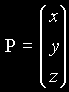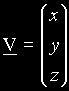3D Geometry Primer: Chapter 1 - Appendix by (14 August 2000) Return to The Archives
 0. Appendix: List of used symbolsThis is a list of the used symbols in this column. You don't have to understand everything right now. Most things will be explained in the different chapters. Real values: a, b, c, ... small, italic letters are used to indicate values. r, s, t These letters are often used for parameters (e.g. parameter representation of a line, of a plane) alpha, beta, gamma, delta, theta, phi, psi, ... small greek "letters" are used for the real value of a angle. pi 3.141592654 = 4*atn(1) –Ą, +Ą negative and positive infinity. If no sign is used, then the positive one is ment. (If hope that the symbol you see is a flat eight. Otherwise, the symbol you see now, will be infinite for you) Indices: a, a, a, ... , a[n], ... if indices are needed and those indices can be ciphers and letters as well, we will write it between square brackets a0, a1, a2, a3, ... If the indices can only be ciphers, then we'll often leave the brackets. a', a", a° If we won't use ciphers too, we can use those little symbols. Real arithmetic +, – addition, subtraction or negation *, / multiplication, division ^, sqrt(r) exponentation, square root of r div, mod integer division, modulo arithmetic abs(r) or |r| absolute value of r: abs(r)=–r if r < 0 abs(0)= 0 abs(r)= r if r > 0 sgn(r) sign of r: sgn(r)=–1 if r < 0 sgn(0)= 0 sgn(r)= 1 if r > 0 sin(theta), cos(theta), tan(theta) sine of theta, cosine or theta, tangent of theta asn(r), acs(r), atn(r) inverse sine of r, inverse cosine of r, inverse tangent of r exp(r), ln(r) natural exponentation of r, natural logarithm of r exp(1)=2.718281828, ln(2.71828182)=1 Points: A, B, C, ... Capitals are used to indicate geometric points. Those points can be 1D, 2D, 3D, ... nD A(a1, a2, a3) B(b1, b2) That's how we write down the coordinates of a point: the x, y, z-values are indicated by the small, italic letter with indices 1,2,3 respective. O O(0,0,0) The origin P or Q P(x,y,z),The letter P often indicates a general point. If we have a certain situation and we say "Let's take a general, random point", then we will call it P. Sometimes the Q is used too. This is if we need 2 general points, or if we can't use the P for some reason. Of course, a general point has general coordinates called x, y and z. P, P, P, ..., P[n], ... P0, P1, P2, ..., Pn, ... P', P", P° Points with indices. Note that we can use letters without brackets here, because we can see the difference between the uppercase point and the lowercase index X, Y, Z X(Ą,0,0), Y(0,Ą,0), Z(0,0,Ą) If you are standing in the origin O, and you follow the x,y,z-axes whole the way into the infinity, that's where you will find the points X,Y,Z I, J, K I(1,0,0), J(0,1,0), K(0,0,1) I, J and K are the unit points on the x,y,z-axes. Notice the similarity between the unit points and the base vectors I, J and K Vectors: AB This is a vector explicit between the points A and B. Notice the underlining! OC C C(c1,c2,c3) Normally, we represent the free vectors with their tail (starting point) in the origin. So we only need to write down the head, possible with it coordinates O O(0,0,0) The null vector V (or U) V(x,y,z),A general vector V, V, V, ... , V[n], ... V0, V1, V2, ..., Vn, ... V', V", V° Vectors with indices. Note that we can use letters without brackets here too. X, Y, Z X(Ą,0,0), Y(0,Ą,0), Z(0,0,Ą) These are the vectors that start in the origin and reach into infinity along the axes. I, J, K I(1,0,0), J(0,1,0), K(0,0,1) The unit vectors or base vectors. Vector arithmetic: ||V|| magnitude of a vector V = length of V = modulus of V = norm of V arg(V) argument of a 2D vector V. Note this is 2D only! r*V scalar product of a value r and a vector U V·U dot product of 2 vectors V and U V×U cross product of 2 vectors V and U Vector bases: B Each set of linear independent vectors that create each other vector of the space with a linear combination of its elements, is called a base. We write down a base with a italic capital. B{I,J,K} A base with its elements: the base vectors. Important note: As you can see, the notation of vectors that start in the origin have much in common with single points. That's why we will handle points like vectors that start in the the origin. The point itself is then the head of the vector. So, now the point P can be treat as the vector OP = P. That will gives us the possibility to use vector arithmetic on points. This similarity and the vector arithmetic will be the subject of Chapter I. Lines: a, b, c, ... Normal, small letters are used to indicate lines. AB Through 2 different points A and B goes exactly 1 line, so you can also describe a line by those two points. u a general line u, u, u, ... , u[n], ... u0, u1, u2, ... u', u", u° lines with indices. Note that we can't use letters without brackets here. x, y, z OX, OY, OZ (sometimes: OI, OJ, OK) The 3 axes are called the x-, y- and z-axes. Because they go through the origin and the infinite X, Y and Z points, you can also write them like OX, OY and OZ. You can also say that they go through the origin and then unit points I, J and K and write them that way (OI, OJ and OK), but we normally don't do that. Planes: ABC Through 3 different points A, B and C (that not are on 1 line!), goes exactly 1 plane, so you can describe a plane by those points. That will be the most common way. OXY, OXZ, OYZ These are the 3 "zero" planes. OXY goes through the points O, X and Y. And is the plane for which z=0 (this is why it is called a zero plane). OXZ is the plane for which y=0, and for OYZ is x=0 pl(N,d) It is also possible to represent a plane by its cartesian equation N·P–d=0, where N is the normal vector and d the distance to the origin. Then, each plane is marked by its N and d, so we can use that to write down a plane. N Sometimes we want to indicate a plane with only 1 letter. Then we will use the normal vector, and show it italic to make the difference. Notice that this is not a common way, and we'll try to not use this. Alfa, Beta, Gamma, Delta, Theta, Phi, Psi, ... Normally if we want to use 1 only letter, we'll use a capital greek letter (Except for alfa and beta, because that is A en B. Use the small greek letters instead). But because we have to write those letters full out, we normally won't use it. Shapes: C(M, r) A circle with middle point M and radius r. Notice that the C is bold. S(M, r) A sphere with middle point M and radius r. Notice that the S is bold too. Other Stuff: // parallelism: e.g. a//b: lines a and b are parallel, U//V: vectors U and V have the same direction. ^ perpendicular to...: e.g. a^b: line a is perpendicular to line b.